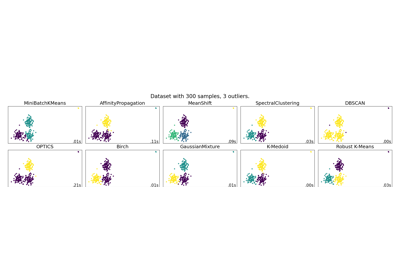# `sklearn_extra.robust`.RobustWeightedKMeans¶

class `sklearn_extra.robust.``RobustWeightedKMeans`(n_clusters=8, weighting='huber', max_iter=100, eta0=0.01, c=None, k=0, kmeans_args=None, tol=0.001, n_iter_no_change=10, verbose=0, random_state=None)[source]

Algorithm for robust kmeans clustering using reweighting algorithm.

This model uses iterative reweighting of samples to make a regression or classification estimator robust.

The principle of the algorithm is to use an empirical risk minimization principle where the risk is estimated using a robust estimator (for example Huber estimator or median-of-means estimator), . The idea behind this algorithm was mentioned before in . This idea translates in an iterative algorithm where the sample_weight are changed at each iterations and are dependent of the sample. Informally the outliers should have small weight while the inliers should have big weight, where outliers are sample with a big loss function.

This algorithm enjoy a non-zero breakdown-point (it can handle arbitrarily bad outliers). When the “mom” weighting scheme is used, k outliers can be tolerated. When the “Huber” weighting scheme is used, asymptotically the number of outliers has to be less than half the sample size.

Read more in the User Guide.

Parameters
weightingstring, default=”huber”

Weighting scheme used to make the estimator robust. Can be ‘huber’ for huber-type weights or ‘mom’ for median-of-means type weights.

max_iterint, default=100

Maximum number of iterations. For more information, see the optimization scheme of base_estimator and the eta0 and burn_in parameter.

eta0float, default=0.01

Constant step-size used during the burn_in period. Used only if burn_in>0. Can have a big effect on efficiency.

cfloat>0 or None, default=None

Parameter used for Huber weighting procedure, used only if weightings is ‘huber’. Measure the robustness of the weighting procedure. A small value of c means a more robust estimator. Can have a big effect on efficiency. If None, c is estimated at each step using half the Inter-quartile range, this tends to be conservative (robust).

kint < sample_size/2, default=1

Parameter used for mom weighting procedure, used only if weightings is ‘mom’. 2k+1 is the number of blocks used for median-of-means estimation, higher value of k means a more robust estimator. Can have a big effect on efficiency. If None, k is estimated using the number of points distant from the median of means of more than 2 times a robust estimate of the scale (using the inter-quartile range), this tends to be conservative (robust).

kmeans_argsdict, default={}

arguments of the MiniBatchKMeans base estimator. Must not contain batch_size.

tolfloat or None, (default = 1e-3)

The stopping criterion. If it is not None, training will stop when (loss > best_loss - tol) for n_iter_no_change consecutive epochs.

n_iter_no_changeint, default=10

Number of iterations with no improvement to wait before early stopping.

verbose: int, default=0

If >0 will display the (robust) estimated loss every 10 epochs.

random_stateint, RandomState instance or None, optional (default=None)

The seed of the pseudo random number generator to use when shuffling the data. If int, random_state is the seed used by the random number generator; If RandomState instance, random_state is the random number generator; If None, the random number generator is the RandomState instance used by np.random.

Notes

One may need to use RobustScaler as a preprocessing.

References

 Guillaume Lecué, Matthieu Lerasle and Timothée Mathieu.

“Robust classification via MOM minimization”, Mach Learn 109, (2020). https://doi.org/10.1007/s10994-019-05863-6 (2018). arXiv:1808.03106

 Christian Brownlees, Emilien Joly and Gábor Lugosi.

“Empirical risk minimization for heavy-tailed losses”, Ann. Statist. Volume 43, Number 6 (2015), 2507-2536.

 Stanislav Minsker and Timothée Mathieu.

“Excess risk bounds in robust empirical risk minimization” arXiv preprint (2019). arXiv:1910.07485.

Examples

```>>> from sklearn_extra.robust import RobustWeightedKMeans
>>> from sklearn.datasets import make_blobs
>>> import numpy as np
>>> rng = np.random.RandomState(42)
>>> X,y = make_blobs(n_samples=100, centers=np.array([[-1, -1], [1, 1]]),
...                  random_state=rng)
>>> km = RobustWeightedKMeans()
>>> _ = km.fit(X)
>>> score = np.mean((km.predict(X)-y)**2)
```
Attributes
cluster_centers_ndarray of shape (n_clusters, n_features)

Coordinates of cluster centers. If the algorithm stops before fully converging (see `tol` and `max_iter`), these will not be consistent with `labels_`.

labels_ndarray of shape (n_samples,)

Labels of each point

inertia_float

Sum of squared distances of samples to their closest cluster center.

n_iter_int

Number of iterations run.

base_estimator_object,

The fitted base_estimator.

weights_array like, length = n_sample.

Weight of each sample at the end of the algorithm. Can be used as a measure of how much of an outlier a sample is.

`__init__`(n_clusters=8, weighting='huber', max_iter=100, eta0=0.01, c=None, k=0, kmeans_args=None, tol=0.001, n_iter_no_change=10, verbose=0, random_state=None)[source]

Initialize self. See help(type(self)) for accurate signature.

## Examples using `sklearn_extra.robust.RobustWeightedKMeans`¶